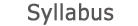2019 - 2020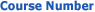0509-1645-03
Ordinary Differential Equations for Mechanical Engineering
FACULTY OF ENGINEERING | DEPARTMENTS OF ENGINEERING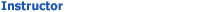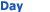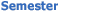Mattan Shaham Wolfson - Engineering 438 Sun 1400-1500 Sem  2
Course description

Credit Points: 3.5
Prerequisites: Differential and Integral Methods, Linear Algebra
Examples from mechanics and electricity of problems involving initial or boundary conditions. First order equations, the existence and uniqueness theorem. Second order linear equations; homogeneous equations and linear independence, the wronksian and lowering the order of an equation, homogeneous equations with constant coefficients. Separation to a homogeneous and an inhomogeneous problem, the method of undetermined coefficients and the method of variation of parameters. One sided Green’s function for solving initial value problems. Reaction to constraints and to initial/boundary conditions. Generalization to nth order equations, the case of constant coefficients. Euler’s formula, series solutions (Frobenius method), Bessel’s function, Legendre’s function, Hermite’s function, Laguerre’s function, regular and singular solutions. The Laplace transform and its applications for solving differential equations, initial and final value theorems, transforms of convolutions. System of first order linear equations. Sturm-Liouville and self-adjoint problems, eigenfunctions and eigenvalues, oscillation of inhomogeneous equations by expansion in eigenfucntions in L2(R), uniform convergence of the expansion, the example of Fourier series.

accessibility declaration